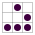# D码点评:9 卡片代码

~名片光线跟踪

``````#include <stdlib.h>   // card > aek.ppm
#include <stdio.h>
#include <math.h>
typedef int i;typedef float f;struct v{
f x,y,z;v operator+(v r){return v(x+r.x
,y+r.y,z+r.z);}v operator*(f r){return
v(x*r,y*r,z*r);}f operator%(v r){return
x*r.x+y*r.y+z*r.z;}v(){}v operator^(v r
){return v(y*r.z-z*r.y,z*r.x-x*r.z,x*r.
y-y*r.x);}v(f a,f b,f c){x=a;y=b;z=c;}v
operator!(){return*this*(1/sqrt(*this%*
this));}};i G[]={247570,280596,280600,
249748,18578,18577,231184,16,16};f R(){
return(f)rand()/RAND_MAX;}i T(v o,v d,f
&t,v&n){t=1e9;i m=0;f p=-o.z/d.z;if(.01
<p)t=p,n=v(0,0,1),m=1;for(i k=19;k--;)
for(i j=9;j--;)if(G[j]&1<<k){v p=o+v(-k
,0,-j-4);f b=p%d,c=p%p-1,q=b*b-c;if(q>0
){f s=-b-sqrt(q);if(s<t&&s>.01)t=s,n=!(
p+d*t),m=2;}}return m;}v S(v o,v d){f t
;v n;i m=T(o,d,t,n);if(!m)return v(.7,
.6,1)*pow(1-d.z,4);v h=o+d*t,l=!(v(9+R(
),9+R(),16)+h*-1),r=d+n*(n%d*-2);f b=l%
n;if(b<0||T(h,l,t,n))b=0;f p=pow(l%r*(b
>0),99);if(m&1){h=h*.2;return((i)(ceil(
h.x)+ceil(h.y))&1?v(3,1,1):v(3,3,3))*(b
*.2+.1);}return v(p,p,p)+S(h,r)*.5;}i
main(){printf("P6 512 512 255 ");v g=!v
(-6,-16,0),a=!(v(0,0,1)^g)*.002,b=!(g^a
)*.002,c=(a+b)*-256+g;for(i y=512;y--;)
for(i x=512;x--;){v p(13,13,13);for(i r
=64;r--;){v t=a*(R()-.5)*99+b*(R()-.5)*
99;p=S(v(17,16,8)+t,!(t*-1+(a*(R()+x)+b
*(y+R())+c)*16))*3.5+p;}printf("%c%c%c"
,(i)p.x,(i)p.y,(i)p.z);}}
``````

``````c++ -O3 -o card card.cpp
./card > card.ppm
``````• 世界由精心组织的球体构成
• 存在指定样式的地板和质感
• 天空有很好的颜色梯度
• 阴影是柔的
• 妈呀,还必须有景深的模糊梯度!

❤共同期待❤

# 筹备活动 ~~~~~~~~~~~~~~~~~~~~~~~~~~~~~~~~~~~~~

## PyCon2013CHina 珠海场

• Python 年度大会
• Pythonner 大趴
• Pythonista 相亲集会
• Pythonic 体验交流

## 巡阅

2013-10-29

### 加入 珠海GDG

1. 注册 Meetup
2. 关注 Zhuhai GDG
3. 或扫描:```    第一时间获知谷歌最新技术,
可以学到如何去谷歌平台上赚钱的思路和方法,
可以认识很多有可能将来一起走上自己创业道路的人,
可以学会把你的创新带向国际市场,
参加那里的活动有经常和国际上的开发者们进行交流的机会...
```

#### PPS:

```0. 有趣 ~ 至少是自个儿有兴趣的领域吧...
1. 有料 ~ 至少有点儿原创的东西吧..
2. 有种 ~ 至少不能是成功学吧!
```

(发空邮件到 [email protected] 即完成订阅)

### 微信公号

```    G术图书 (gb:推荐好书,书无中外)
D码点评 (dd:麻辣评点,善意满盈)
G说公论 (gt:时评杂文,新旧不拘)
珠的自白(dm:大妈自述,每周一篇)
海选文章(hd:得要相信,大妈法眼)
```

#### 订阅方法

• 搜索微信号 `GDG-ZhuHai`
• 或查找公众号: `GDG珠海`
• 或扫描:### GDG珠海 社区资源:

#### 自怼圈/年度番新### 蟒营®编程思维提高班Python版

**2021.01.11** 因大妈再次创业暂停定期开设, 转换为预约触发:

#### 追问

[email protected]